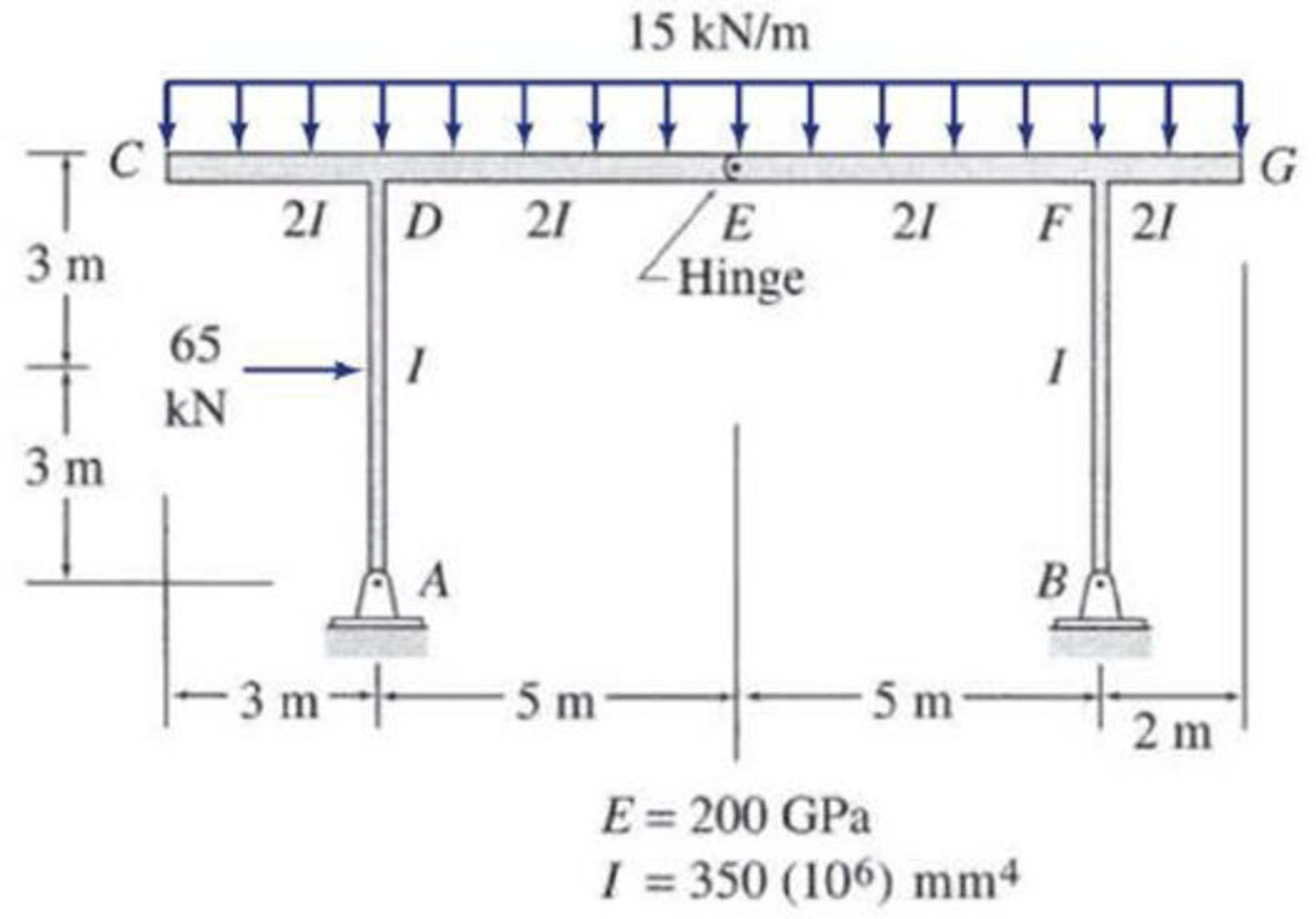# Use the virtual work method to determine the rotation of joint D of the frame shown. FIG P7.49, P7.50

#### Solutions

Chapter
Section
Chapter 7, Problem 49P
Textbook Problem
481 views

## Use the virtual work method to determine the rotation of joint D of the frame shown.FIG P7.49, P7.50

To determine

Find the rotation at joint D of the frame using virtual work method.

### Explanation of Solution

Given information:

The frame is given in the Figure.

The value of E is 200 GPa and I is 350×106mm4.

Apply the sign conventions for calculating reactions, forces and moments using the three equations of equilibrium as shown below.

• For summation of forces along x-direction is equal to zero (Fx=0), consider the forces acting towards right side as positive (+) and the forces acting towards left side as negative ().
• For summation of forces along y-direction is equal to zero (Fy=0), consider the upward force as positive (+) and the downward force as negative ().
• For summation of moment about a point is equal to zero (Matapoint=0), consider the clockwise moment as negative and the counter clockwise moment as positive.

Calculation:

Consider the real system.

Draw a diagram showing all the given real loads acting on it.

Let the bending moment due to real load be M.

Sketch the real system of the frame as shown in Figure 1.

Find the reactions at the supports A and B:

Summation of moments about A is equal to 0.

MA=0By(10)15(15)(4.5)65(3)=0By=120.75kN

Summation of forces along y-direction is equal to 0.

+Fy=0Ay+By15(15)=0Ay+120.7515(15)=0Ay=104.25kN

Consider ACDE, summation of moments about E is equal to 0.

ME=015(8)(4)+65(3)Ax(6)Ay(5)=0480+195Ax(6)104.25(5)=06Ax+153.75=0Ax=25.625kN

Summation of forces along x-direction is equal to 0.

+Fx=0AxBx+65=025.625Bx+65=0Bx=39.375kN

Sketch the member end forces of the real system as shown in Figure 2.

Consider the virtual system.

Draw a diagram of frame without the given real loads. For rotation apply unit couple at the joint in the desired direction.

Let the bending moment due to virtual couple be Mv.

Sketch the virtual system of the frame with unit couple at joint D as shown in Figure 3.

Find the reactions at the supports A and B:

Summation of moments about A is equal to 0.

MA=0By(10)1=0By=110kN

Summation of forces along y-direction is equal to 0.

+Fy=0Ay+110=0Ay=110kN

Consider ACDE, summation of moments about E is equal to 0.

ME=01+Ax(6)+Ay(5)=01+Ax(6)+110(5)=06Ax=112

6Ax=12Ax=112kN

Summation of forces along x-direction is equal to 0.

+Fx=0AxBx=0112Bx=0Bx=112kN

Sketch the member end forces of the virtual system as shown in Figure 4.

Find the equations for M and Mv for the 4 segments of the frame as shown in Table 1.

 Segment x-coordinate M (kN⋅m) Mv (kN⋅m) Origin Limits (m) AH A 0−3 25.625x −x12 HD A 3−6 25.625x−65(x−3) −x12 BF B 0−6 39.375x x12 DF D 0−10 59.25x−108.75−7.5x2 12−x10

Find the slope at D using the virtual work expression:

θD=0LMvMEIdx (1)

Moment of inertia of span AH is I, moment of inertia of span HD is I, moment of inertia of span BF is I, and moment of inertia of span DF is 2I.

Rearrange Equation (1) for the limits 03, 36, 06, and 010 as follows.

θD=1EI[03(MvM)dx+36(MvM)dx+06(MvM)dx+12010(MvM)dx]

Substitute x12 for Mv, 25

### Still sussing out bartleby?

Check out a sample textbook solution.

See a sample solution

#### The Solution to Your Study Problems

Bartleby provides explanations to thousands of textbook problems written by our experts, many with advanced degrees!

Get Started

Find more solutions based on key concepts
Calculate the second moment of area of a 4 in. diameter shaft about the xx and yy axes, as shown.

Engineering Fundamentals: An Introduction to Engineering (MindTap Course List)

Why is it important to deliver effective project reports and communications?

Systems Analysis and Design (Shelly Cashman Series) (MindTap Course List)

Describe the uses of tags. List steps to tag digital content.

Enhanced Discovering Computers 2017 (Shelly Cashman Series) (MindTap Course List)

Abrasive machining makes use of ______ to remove material.

Precision Machining Technology (MindTap Course List)

Why is a slight forward torch angle helpful for cutting?

Welding: Principles and Applications (MindTap Course List)

If your motherboard supports ECC DDR3 memory, can you substitute non-ECC DDR3 memory?

A+ Guide to Hardware (Standalone Book) (MindTap Course List)